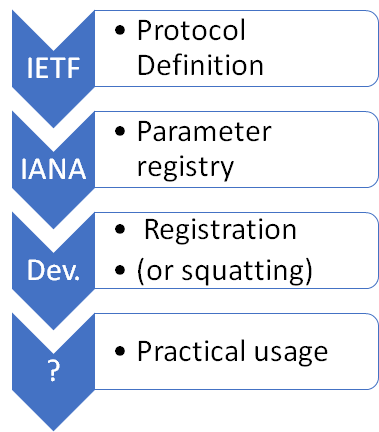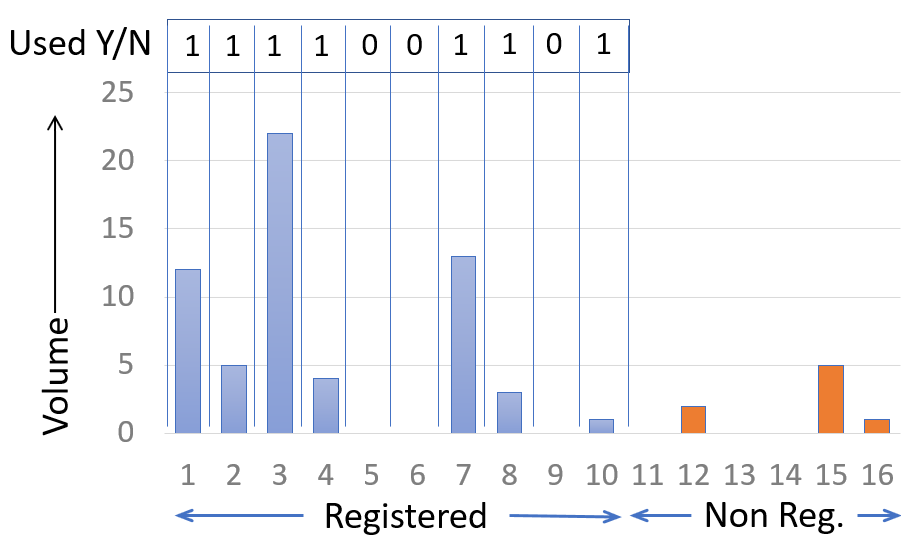# ITHI Metric M6, IANA registries for DNS parametersThe IANA manages a set of parameter registries on behalf of the IETF. These registries are requested by the IETF, as part of a protocol definition. The IANA will then establish the corresponding registry. Developers are then supposed to register new values when the need arises. For example, developers who find the need for a new DNS Record Type will document that new type in an Internet Draft, and get the new type registered by IANA in the DNS RR Type registry. Of course, there is a risk that some developers will find that process too cumbersome, and will instead use an unregistered value, a process that we call “squatting”. The goal of the M6 metrics is to measure if registered values are used, and whether we observe squatting. We want to tract this squatting and usage over time, and we also want to track the usage level of specific parameters.

We compute the M6 metric for three groups of registries managed by IANA: the Domain Name System (DNS) Parameters, the Domain Name System Security (DNSSEC) Algorithm Numbers, and the DNS-Based Authentication of Named Entities (DANE) Parameters. Each of these groups includes different parameter sets. We will track the health of each of these parameters by a set of metrics of the form “M6.N.X.*”, where “M6.N.X” is the metric index composed of the registry name “N” and the parameter set index “X”. These three groups include the following set of parameters:

GroupParametersMetric Index
DNSDNS CLASSesM6.DNS.1
Resource Record (RR) TYPEsM6.DNS.2
DNS OpCodesM6.DNS.3
DNS RCODEsM6.DNS.4
AFSDB RR SubtypeM6.DNS.5
DHCID RR Identifier Type CodesM6.DNS.6
DNS Label TypesM6.DNS.7
DNS EDNS0 Option Codes (OPT)M6.DNS.8
EDNS version Number (8 bits)M6.DNS.11
Child Synchronization (CSYNC) Flags M6.DNS.12
DNSSECDNS Security Algorithm NumbersM6.DNSSEC.1
DNS KEY Record Diffie-Hellman Prime LengthsM6.DNSSEC.2
DNS KEY Record Diffie-Hellman Well-Known Prime/Generator PairsM6.DNSSEC.3
DANETLSA Certificate UsagesM6.DANE.1
TLSA SelectorsM6.DANE.2
TLSA Matching TypesM6.DANE.3

Each of this set of parameters is associated with a metric index. For each of these indices, we will compute three metrics:

Metric NumberMetric nameMetric definition
M6.X.N.1Parameter usage for table of metric N in group X Number of parameters found at least once in real traffic, divided by the total number of parameters found registered in the table.
M6.X.N.2Squat rate for table of index N in group X Total number of instances of unregistered parameters found in real traffic, divided by the total number of parameter instances found in real traffic.
M6.X.N.3.

Usage level of registered parameter P in table of index N in group X Number of instances of the registered parameter in real traffic.The computation of the usage and squatting metrics (M6.X.N.1 and M6.X.N.2) can be explained by using as example a fictitious registry that could manage a total of 16 values. In our example, values 0 to 10 have been properly registered. Values 12, 15 and 16 are not registered, but they do appear in some the traces.

To compute the “usage” metric, we look at the traffic observed for the registered values. We see some traffic for values 1, 2, 3, 4, 7, 8 and 10, and mark a “1” in the corresponding “Used Y/N” table; there is no traffic for the values 5, 6, and 9, so we mark a “0” in the table. If we sum the ones, we get 7 registered parameters out of 10. The usage metric is thus:

• Usage = number used / number registered = 7/10 = 70%.
To compute the “squatting” metric, we compute the total volume of traffic for the squatted values 12, 15 and 16, which in your example is 8 units. The total traffic for all the registered value in our example is 60 units. The squatting metric is thus:
• Squatting = volume non registered/volume total = 8 / (60 + 8) = 11.8%

The current values of these numbers are displayed here, using the definitions that we presented above.